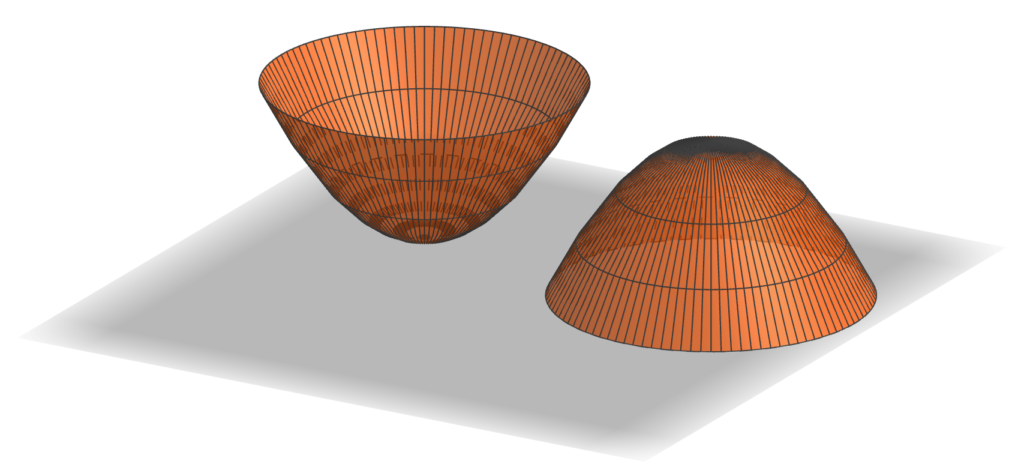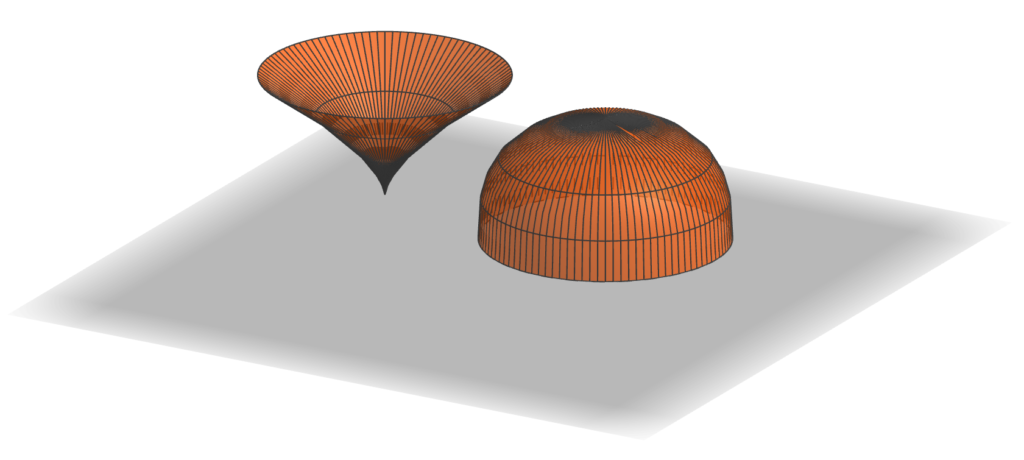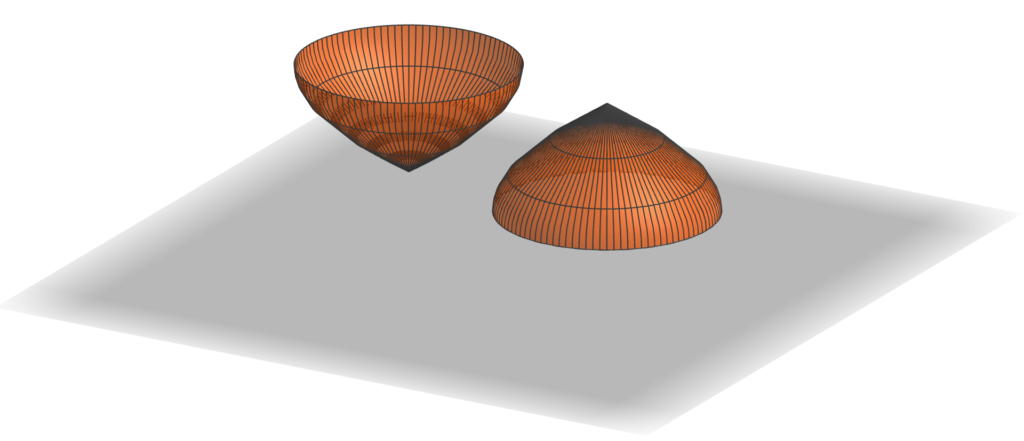10 Apr

So I had zeroed in on the proof of the Archimedean volume relationship as the source of my giveaway for G4G13. But how to create an interesting variation?
The first thing to notice is that the function of h that appears in the proof in the cross-sectional area of of the cone, namely h², could be any function f(h) whatsoever, and the proof would be just as valid — solids where the cross-sectional areas went as f(h) and 1 – f(h) would have volumes that add up to that of a cylinder.

So, in some sense the simplest example would be solids with cross-sectional area going as h and 1-h. What would they look like? Well, they would correspond to radii at height h of √h and √(1-h). In other words, they would be solids of revolution about the z-axis of the graphs of √h and √(1-h), like so:First, these two objects look identical (just one is upside down as compared to the other). But that’s no surprise: as h goes from 0 to 1, 1-h goes from 1 to 0, and at the same rate. But that means that each one must have half the volume of the corresponding cylinder. And further, since the graph of √h is a parabola (on its side), these objects are paraboloids. So we’ve established

the paraboloid of unit height and radius has half the volume of the cylinder with unit height and radius,

which is kind of cool already. So should this be the giveaway?

It was time to figure out what the giveaway items would actually be. Since the principle was about volumes, they needed to be something which was related to volume, but I didn’t want to create another hourglass. So the obvious answer was that they should be measuring cups. And that decision suggested that I shouldn’t use f(h) = h, since two identical measuring cups would be boring.

Since I’d now looked at h and h², the natural next choice would be h³. Here’s what the corresponding solids look like:Those look pretty interesting. So if I used these shapes, what would the volumes be? Now it’s by far easiest to go ahead and use that late-17th-century invention, calculus. The volume of the “pointy” shape should be $\int_0^1 \pi h^3 dh = \pi \left.h^4/4 \right|_0^1 = \pi/4$. In other words, if the cylinder were scaled to have a volume of one cup, say, then the pointy shape would have a volume of 1/4 cup and the rounded one would have a volume of 3/4 cup. That seemed pretty good: since most measuring cup sets do not include one with volume 3/4 cup, the resulting set might actually be useful.

But there’s another place you can vary the construction in the proof: the shape of the cross-sections. There’s no requirement that they be circles; you can scale any shape, and the overall volume will scale by the area of the largest cross section. (That’s the $\pi$ in the above formulas, namely the area of the top circle of the pointy shape or the bottom circle of the rounded one.) So I decided to use this flexibility to create one more link to G4G13: make the cross section of the “cylinder” be a regular 13-gon, the 3/4-cup measure a regular octagon, and the quarter-cup measure be a regular pentagon. These shapes echo the Fibonacci decomposition 13 = 8 + 5 where the mathematical free association began.

So although that brings us to the shapes that actually went into the G4G13 giveaway, this exploration wouldn’t be complete without emphasizing that there’s no reason f(h) has to be a power of h at all. We’re literally free to use any function at all, so long as it takes values between 0 and 1. In particular, why not try the most famous pair of functions that sum to 1 for the cross-sectional areas of the two cups, namely cos²(h) and sin²(h)? That would produce these shapes:As in the first case, the shapes are identical, although in this case because of the identity sin(x) = cos(π/2 – x). So again, this shape has half the volume of the corresponding cylinder, even though it is clearly not a paraboloid (as you can see, for example, by the fact that it comes to a point at its apex; a paraboloid is smooth there). We could spend forever exploring different combinations of functions that would give Archimedes-like decompositions of a cylinder, but it’s time to turn attention to fabricating the G4G13 giveaway.